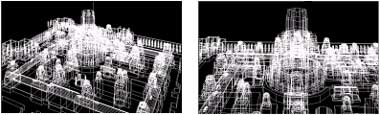Home Articles PCA colour image compression using vector quantization

# PCA colour image compression using vector quantization

ACRS 1999

Poster Session 5

PCA Colour Image Compression Using Vector Quantization

S. Chitwong(1), A. somboonkaew(1,2), F. cheevasuvit(1), K.Dejhan(1) and s. Mitatha(1)
(1)Faculty of Engineering, King Mongkut’s Institute of Technology ladkraband,
Bangkok 10520, Thailand
(2)Electro-Optics Lab, in Faculty of Science, King Mongkut’s Institute of Technology
ladkrabang, National Electronics and Computer Technology
Bangkok 10520, Thailand

Abstract
Since the method of principal components analysis (PCA) is a powerful and efficient method for dimensionality reduction. Therefore, the generated colour image from the first three principal components can be contained almost the total variance of the original images. So many applications can be accomplished by using the PCA colour image. Generally, the PCA colour image needs 24 bits to represent the colour of each pixel. This will cause the problems of memory storage and transmitting channel bandwith. To conquer these problems, this paper proposes the vector quantization technique to compress the PCA image. Only 8 bits are used to encode the PCA colour image instead of 24 bits. The initial 256 code vectors in the codebook are selected from the 3-dimensional cluster diagram of first three components.

1.Introduction
principal components analysis (PCA) is first presented by H. Hotelling , hence sometimes, it is called Hotelling transform. PCA Plays and important role in the remote sensing applications such as change detection, classification, enhancement and data reduction. The PCA technique is based on the assumption that the variance of image data may be used as a measure of that image’s 10 information content . The satellite data acquisition system compose of many spectral sensors. Since each object can be well reflected in different spectral bands. In order to discriminate the object of interest, therefore, many spectral images are integrated to consider in the same time. PCA algorithm can be served this requirement because it can agglomerate the almost the total information of original images in the first three principal components which have the higher variance. Then the PCA colour image, obtained by the first three principal components, Will be used in image interpretation and classification. However, a colour image is required 24 bits for pixel encoding. This will cause the problems of bandwidth capacity and data storage. To carry out these problems, the data compression must be applied. As the vector quantization is quite efficacious method for data compression, then it is selected to compress the PCA colour image. Only 256 code vectors are selected in the codebook of vector quantization method. The index of code vector is encoded by 8 bits. Therefore, we can reduce the number of bits by three times.

2. PCA algorithm
PCA is a linear transform that can be written asX is a p-dimensional of original variable
The expected value of Y is obtained by
E(Y) = E(CX +B)
=CE(X) +B (2)
=Cmx + B = my
Normally, it may be chosen my = 0, as
Cmx +B = 0
-Cmx = B (3)

By substituting equation (3) into equation (1),
Y = CX – Cmx
Y = C(X – mx)

ACRS 1999

Poster Session 5

PCA Colour Image Compression Using Vector Quantization

But my = 0, the covariance of Y is then

E(YYT) = L = E{[C(X-mx)][C(X-mx)]T}
L = CE[(X-mx) (X-mx)T]CT (4)
L = C